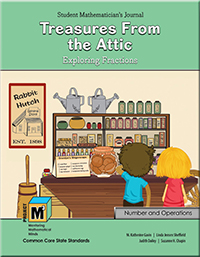# Project M³ Curriculum Units — Level 4-5

## Treasures From the Attic: Exploring Fractions

(get the book choose grades 4-5)In this unit students are introduced to two children, Tori and Jordan. In their grandparents’ attic Tori and Jordan uncover hidden treasures from a general store that their grandmother’s grandparents used to own. These treasures lead to an interesting exploration of fraction concepts. The focus of the entire unit is on making sense of fractions rather than on learning algorithms to perform computations. This is a significant departure from more traditional approaches. It is important for students to think about and picture the relative sizes of fractions and make estimates based on their mathematical thinking when ordering, comparing, adding, subtracting, multiplying or dividing two or more fractions. Since fractions are such an important part of our everyday experiences, focusing on meaning rather than rules actually gives students a facility for understanding and working with fractions that will benefit them throughout their lives. This is in line with the research guiding and supporting the Common Core Standards for Mathematics as outlined in the Progressions document, Numbers and Operations – Fractions that can be found at ime.math.arizona.edu/progressions/.

In the first chapter, students are exposed to a variety of models (specifically set, linear and area models) to name equivalent fractions. Using a variety of models helps students gain a firm grasp of equivalence, and this, in turn, enables them to generalize and then to apply their understanding to comparing and ordering fractions. When comparing and ordering fractions, students also learn to use multiple strategies to analyze the size of fractions, such as common numerators, common denominators, benchmarks and missing parts of the whole. Students also investigate the density of the real number system as they learn that between any two fractions, another one can always be found. This implies that there are no holes or gaps in the real number line. They also discover something quite exciting—that there is an infinite number of fractions equivalent to any given fraction!

In working with addition, subtraction, multiplication and division of fractions in the second chapter, again the focus is on the meaning of mathematical operations as they are used with fractions in a variety of contextual situations. In this chapter, as they solve problems in different situations, students first construct and discuss their own methods for adding and subtracting fractions of all sizes before they are introduced to the common algorithms. Students initially use the physical models, drawings and equivalent fraction charts that they used in the first chapter to develop written algorithms for the operations. After discussing and comparing their own methods, they then compare their methods to the common algorithms and decide which are most meaningful and efficient. This is expanded to an introduction to multiplication and division of fractions, using everyday experiences such as cooking.